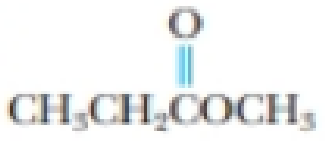Chapter 23, Problem 69PS

Chapter
Section
Textbook Problem

Identify the functional groups in the following molecules.(a) CH3CH2CH2OH(b)(c)(d)(a)

Interpretation Introduction

Interpretation: The functional group in the given molecule has to be identified.

Concept introduction:

Functional group: They are certain substitutes in the organic molecules which are determine the characteristic reactions taking place in it.

Alcohol: It is an organic compound where it contains at least one OH group. It is represented as ROH.

Explanation

The given compound has the molecular formula CH3CH2CH2OH

(b)

Interpretation Introduction

Interpretation: The functional group in the given molecule has to be identified.

Concept introduction:

Functional group: They are certain substitutes in the organic molecules which are determine the characteristic reactions taking place in it.

Amide: One NH2, NHR' or NR'2 group is attached to the carbonyl carbon atom of the compound. It is represented as RCONR'2.

(c)

Interpretation Introduction

Interpretation: The functional group in the given molecule has to be identified.

Concept introduction:

Functional group: They are certain substitutes in the organic molecules which are determine the characteristic reactions taking place in it.

Carboxylic acid: One OH group is attached to the carbonyl carbon atom of the compound. It is represented as RCOOH

(d)

Interpretation Introduction

Interpretation: The functional group in the given molecule has to be identified.

Concept introduction:

Functional group: They are certain substitutes in the organic molecules which are determine the characteristic reactions taking place in it.

Ester: One OR' group is attached to the carbonyl carbon atom of the compound. It is represented as RCOOR'. The hydrogen atom of COOH is replaced by an alkyl group.

Still sussing out bartleby?

Check out a sample textbook solution.

See a sample solution

The Solution to Your Study Problems

Bartleby provides explanations to thousands of textbook problems written by our experts, many with advanced degrees!

Get Started

Find more solutions based on key concepts